# modwpt

Maximal overlap discrete wavelet packet transform

## Syntax

``wpt = modwpt(x)``
``wpt = modwpt(x,wname)``
``wpt = modwpt(x,lo,hi)``
``wpt = modwpt(___,lev)``
``````[wpt,packetlevs] = modwpt(___)``````
``````[wpt,packetlevs,cfreq] = modwpt(___)``````
``````[wpt,packetlevs,cfreq,energy] = modwpt(___)``````
``````[wpt,packetlevs,cfreq,energy,relenergy] = modwpt(___)``````
``[___] = modwpt(___,Name=Value)``

## Description

example

````wpt = modwpt(x)` returns the terminal nodes for the maximal overlap discrete wavelet packet transform (MODWPT) for the 1-D signal, `x`. The output is time-delayed compared to the input signal. For more information, see MODWPT and MODWPT Details.```

example

````wpt = modwpt(x,wname)` returns the MODWPT using the orthogonal wavelet filter specified by the `wname`.```
````wpt = modwpt(x,lo,hi)` returns the MODWPT using the orthogonal scaling filter, `lo`, and wavelet filter, `hi`.```
````wpt = modwpt(___,lev)` returns the terminal nodes of the wavelet packet tree at positive integer level `lev`. ```

example

``````[wpt,packetlevs] = modwpt(___)``` returns a vector of transform levels corresponding to the rows of `wpt`. ```
``````[wpt,packetlevs,cfreq] = modwpt(___)``` returns the center frequencies of the approximate passbands corresponding to the rows of `wpt`.```

example

``````[wpt,packetlevs,cfreq,energy] = modwpt(___)``` returns the energy (squared L2-norm) of the wavelet packet coefficients for the nodes in `wpt`.```

example

``````[wpt,packetlevs,cfreq,energy,relenergy] = modwpt(___)``` returns the relative energy for the wavelet packets in `wpt`.```

example

````[___] = modwpt(___,Name=Value)` specifies options using one or more name-value arguments in addition to the input arguments in previous syntaxes. For example, to return the full wavelet packet tree, set `FullTree` to `true`.```

## Examples

collapse all

Obtain the terminal nodes of the MODWPT of an electrocardiogram (ECG) signal by using `modwpt` with default settings. The signal is sampled at 180 Hz. Because the signal has 2048 elements, by default, the terminal nodes are at level 4.

```load wecg wpt = modwpt(wecg);```

`wpt` is a 16-by-2048 matrix containing the sequency-ordered wavelet packet coefficients for the terminal nodes. Each node corresponds to an approximate passband filtering of $\left[n{f}_{s}/{2}^{5},\left(n+1\right){f}_{s}/{2}^{5}\right)$, where n = 0,...,15, and ${f}_{s}$ is the sampling frequency. Plot the wavelet packet coefficients at node (4,2), which is level 4, node 2.

```plot(wpt(3,:)) axis tight title("Node (4,2) Wavelet Packet Coefficients")```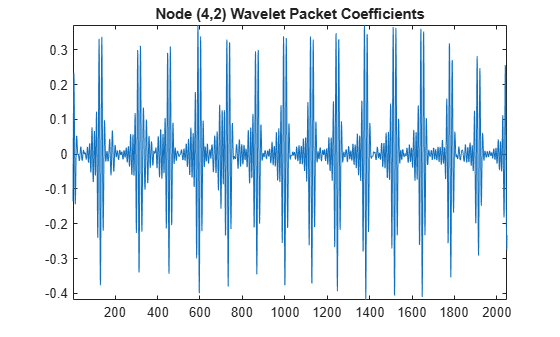Obtain the MODWPT of Southern Oscillation Index data with the Daubechies extremal phase wavelet with two vanishing moments (`'db2'`).

```load soi wsoi = modwpt(soi,"db2");```

Verify that the size of the resulting transform contains 16 nodes. Each node is in a separate row.

`size(wsoi)`
```ans = 1×2 16 12998 ```

Obtain the MODWPT and full wavelet packet tree of an ECG waveform using the default length 18 Fejér-Korovkin (`'fk18'`) wavelet. Extract and plot the node coefficients at level 3, node 2.

```load wecg [wpt,packetlevels,cfreq] = modwpt(wecg,FullTree=true); p3 = wpt(packetlevels==3,:); node=2; plot(p3(node+1,:)) axis tight title("Node (3,2) Wavelet Coefficients")```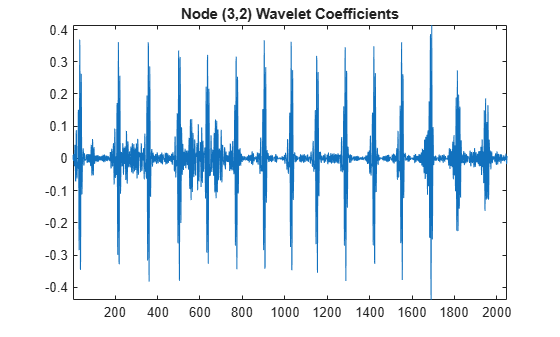Display the center frequencies at level 3.

`cfreq(packetlevels==3,:)`
```ans = 8×1 0.0312 0.0938 0.1562 0.2188 0.2812 0.3438 0.4062 0.4688 ```

Obtain the MODWPT energy and relative energy of an ECG waveform.

```load wecg [wpt,~,cfreq,energy,relenergy] = modwpt(wecg);```

Show that the sum of the MODWPT energies is equal to the total energy in the original signal.

`sum(energy)`
```ans = 298.2759 ```
`sum(wecg.^2)`
```ans = 298.2759 ```

Plot the MODWPT energy by node.

```bar(1:16,energy) xlabel("Node") ylabel("Energy") title("Energy by Node")```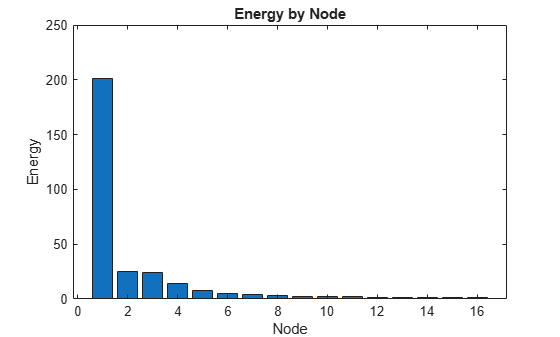Plot the relative energy by node.

```bar(1:16,relenergy*100) xlabel("Node") ylabel("Percent Energy") title("Energy Relative to Signal Energy by Node")```Create a signal consisting of two intermittent sine waves in noise. The sine wave frequencies are 150 Hz and 200 Hz. The data is sampled at 1000 Hz.

```Fs = 1000; t = 0:1/Fs:1-1/Fs; x = cos(2*pi*150*t).*(t>=0.2 & t<0.4)+ ... sin(2*pi*200*t).*(t>0.6 & t<0.9); y = x+0.05*randn(size(t));```

Obtain the default and time-aligned MODWPT of the signal.

```[wptn,~,Fnon] = modwpt(y); [wpta,~,Falign] = modwpt(y,TimeAlign=true);```

Plot the signal. Compare with the default and time-aligned time-frequency plots.

```tiledlayout(3,1) nexttile plot(t,x) title("Signal") ylabel("Amplitude") nexttile contour(t,Fs*Fnon,abs(wptn).^2) grid on ylabel("Hz") title("Time-Frequency Plot") nexttile contour(t,Fs*Falign,abs(wpta).^2) grid on xlabel("Time (s)") ylabel("Hz") title("Time-Frequency Plot (Time-Aligned)")```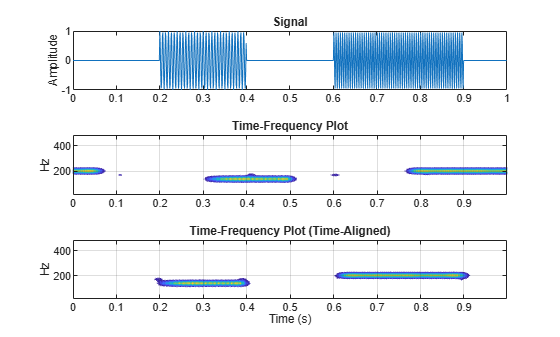## Input Arguments

collapse all

Input signal, specified as a row or column vector. `x` must have at least two elements.

Data Types: `single` | `double`
Complex Number Support: Yes

Analyzing wavelet, specified as a character vector or string scalar. The wavelet must be orthogonal. Orthogonal wavelets are designated as type 1 wavelets in the wavelet manager, `wavemngr`.

Valid built-in orthogonal wavelet families are: Best-localized Daubechies (`"bl"`), Beylkin (`"beyl"`), Coiflets (`"coif"`), Daubechies (`"db"`), Fejér-Korovkin (`"fk"`), Haar (`"haar"`), Han linear-phase moments (`"han"`), Morris minimum-bandwidth (`"mb"`), Symlets (`"sym"`), and Vaidyanathan (`"vaid"`).

For a list of wavelets in each family, see `wfilters`. You can also use `waveinfo` with the wavelet family short name. For example, `waveinfo("db")`. Use `wavemngr("type",wname)` to determine if `wname` is orthogonal (returns 1). For example, `wavemngr("type","db6")` returns 1.

Filters, specified as a pair of even-length real-valued vectors. `lo` is the scaling (lowpass) filter and `hi` is the wavelet (highpass) filter. The filters must satisfy the conditions for an orthogonal wavelet. For more information, see `wfilters` and `isorthwfb`. You cannot specify both `wname` and a filter pair `lo,hi`.

Note

By default, the `wfilters` function returns two pairs of filters associated with an orthogonal or biorthogonal wavelet you specify. To agree with the usual convention in the implementation of `MODWPT` in numerical packages, when you specify an orthogonal wavelet `wname`, the `modwpt` function internally uses the second pair of filters returned by `wfilters`. For example,

```wpt = modwpt(x,"db2");```

is equivalent to

```[~,~,lo,hi] = wfilters("db2"); wpt = modwpt(x,lo,hi);```

This convention is different from the one followed by most Wavelet Toolbox™ discrete wavelet transform functions when decomposing a signal. Most functions internally use the first pair of filters.

Data Types: `single` | `double`

Transform level, specified as a positive integer less than or equal to `floor(log2(numel(x)))`.

### Name-Value Arguments

Specify optional pairs of arguments as `Name1=Value1,...,NameN=ValueN`, where `Name` is the argument name and `Value` is the corresponding value. Name-value arguments must appear after other arguments, but the order of the pairs does not matter.

Example: `wpt = modwpt(x,FullTree=true)` returns the full wavelet packet tree.

Before R2021a, use commas to separate each name and value, and enclose `Name` in quotes.

Example: `wpt = modwpt(x,'FullTree',true)` returns the full wavelet packet tree.

Option to return the full wavelet packet tree, specified as a numeric or logical `1` (`true`) or `0` (`false`). If you specify `false`, then `modwpt` returns only the terminal (final-level) wavelet packet nodes. If you specify `true`, then `modwpt` returns the full wavelet packet tree down to the specified level.

Example: `FullTree=true` specifies to return the full wavelet packets tree.

Option to time align wavelet packet coefficients with signal features, specified as a numeric or logical `1` (`true`) to time align or `0` (`false`) to not align.

The scaling and wavelet filters have a time delay. Circularly shifting the wavelet packet coefficients in all nodes aligns the signal and wavelet coefficients in time. If you want to reconstruct the signal, such as by using `imodwpt`, do not shift the coefficients because time alignment is done during the inversion process.

Example: `TimeAlign=true` specifies to time-align the wavelet packet coefficients with the signal.

## Output Arguments

collapse all

Wavelet packet tree, returned as a matrix with each row containing the sequency-ordered wavelet packet coefficients. By default, `wpt` contains only the terminal level for the MODWPT. The default terminal level is either level 4 or `floor(log2(numel(x)))`, whichever is smaller. At level 4, `wpt` is a 16-by-`numel`(`x`) matrix. For the full tree, at level j, `wpt` is a 2j+2-2-by-`numel`(`x`) matrix, with each row containing the packet coefficients by level and index. The approximate passband for the nth row of `wpt` at level j is $\left[\frac{n-1}{{2}^{\left(j+1\right)}},\frac{n}{{2}^{\left(j+1\right)}}\right)$ cycles/sample, where n = 1,2,...2j.

Transform levels, returned as a vector. The levels correspond to the rows of `wpt`.

• If `wpt` contains only the terminal level coefficients, `packetlevs` is a vector of constants equal to the terminal level.

• If `wpt` contains the full wavelet packet table, `packetlevs` is a vector with 2j elements for each level, j. To select all the wavelet packet nodes at a particular level, use `packetlevs` with logical indexing.

Center frequencies of the approximate passbands in the `wpt` rows, returned as a vector. The center frequencies are in cycles/sample. To convert the units to cycles/unit time, multiply `cfreq` by the sampling frequency.

Energy of the wavelet packet coefficients for the `wpt` nodes, returned as a vector. The sum of the energies (squared L2 norms) for the wavelet packets at each level equals the energy in the signal.

Relative energy for each level, returned as a vector. The relative energy is the proportion of energy in each wavelet packet by level, relative to the total energy of that level. The sum of relative energies in all packets at each level equals 1.

## Algorithms

collapse all

### MODWPT and MODWPT Details

The output of the MODWPT (`modwpt`) is time-delayed compared to the input signal. Most filters used to obtain the MODWPT have a nonlinear phase response, which makes compensating for the time delay difficult. This is true for all orthogonal scaling and wavelet filters, except the Haar wavelet. It is possible to time-align the coefficients with the signal features, but the result is an approximation, not an exact alignment with the original signal. The MODWPT partitions the energy among the wavelet packets at each level. The sum of the energy over all the packets equals the total energy of the input signal. The output of MODWPT is useful for applications where you want to analyze the energy levels in different packets.

The MODWPT details (`modwptdetails`) are the result of zero-phase filtering of the signal. The features in the MODWPT details align exactly with features in the input signal. For a given level, summing the details for each sample returns the exact original signal. The output of the MODWPT details is useful for applications that require time-alignment, such as nonparametric regression analysis.

### Sequency-ordered Wavelet Packet Tree

The `modwpt` function performs a discrete wavelet packet transform and produces a sequency-ordered wavelet packet tree. Compare the sequency-ordered and normal (Paley)-ordered trees. $\stackrel{˜}{G}\left(f\right)$ is the scaling (lowpass) analysis filter, and $\stackrel{˜}{H}\left(f\right)$ represents the wavelet (highpass) analysis filter. The labels at the bottom show the partition of the frequency axis [0,1/2] into subbands.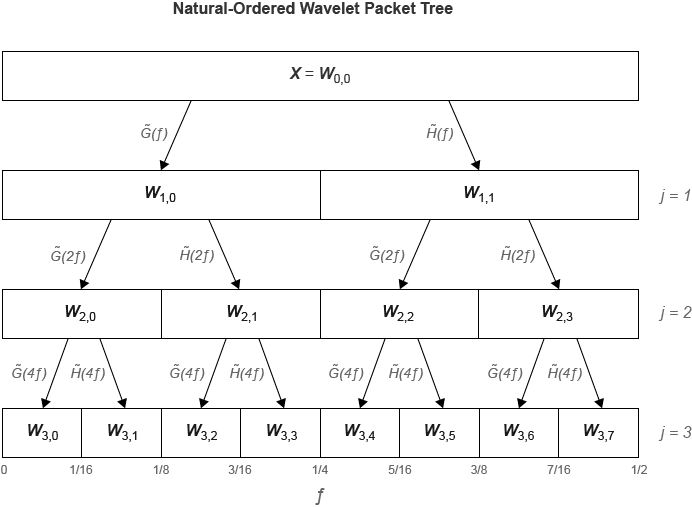Percival, Donald B., and Andrew T. Walden. Wavelet Methods for Time Series Analysis. Cambridge Series in Statistical and Probabilistic Mathematics. Cambridge ; New York: Cambridge University Press, 2000.

 Walden, A. T., and A. Contreras Cristan. “The Phase–Corrected Undecimated Discrete Wavelet Packet Transform and Its Application to Interpreting the Timing of Events.” Proceedings of the Royal Society of London. Series A: Mathematical, Physical and Engineering Sciences 454, no. 1976 (August 8, 1998): 2243–66. https://doi.org/10.1098/rspa.1998.0257.